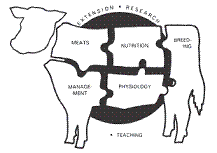## Animal Science Department## Nebraska Beef Cattle Reports

2013

#### Citation

Published in 2013 Nebraska Beef Cattle Report.

#### Abstract

The purpose of beef cattle and beef product research at University of Nebraska–Lincoln is to provide reference information that represents the various populations (cows, calves, heifers, feeders, carcasses, retail products, etc.) of beef production. Obviously, the researcher cannot apply treatments to every member of a population; therefore, he/she must sample the population. The use of statistics allows the researcher and readers of the Nebraska Beef Report the opportunity to evaluate separation of random (chance) occurrences and real biological effects of a treatment. Following is a brief description of the majorstatistics used in the beef report. For a more detailed description of the expectations of authors and parameters used in animal science see Journal of Animal Science Style and Form at: http://jas.fass.org/misc/ifora.shtml.

Mean — Data for individual experimental units (cows, steers, steaks) exposed to the same treatment are generally averaged and reported in the text, tables and figures. The statistical term representing the average of a group of data points is mean.

Variability — The inconsistency among the individual experimental units used to calculate a mean for the item measured is the variance. For example, if the ADG for all the steers used to calculate the mean for a treatment is 3.5 lb then the variance is zero. But, this situation never happens! However, if ADG for individual steers used to calculate the mean for a treatment range from 1.0 lb to 5.0 lb, then the variance is large. The variance may be reported as standard deviation (square root of the variance) or as standard error of the mean. The standard error is the standard deviation of the mean as if we had done repeated samplings of data to calculate multiple means for a given treatment. In most cases treatment means and their measure of variability will be expressed as follows: 3.5 ± 0.15. This would be a mean of 3.5 followed by the standard error of the mean of 0.15. A helpful step combining both the mean and the variability from an experiment to conclude whether the treatment results in a real biological effect is to calculate a 95% confidence interval. This interval would be twice the standard error added to and subtracted from the mean. In the example above, this interval is 3.2-3.8 lb. If in an experiment, these intervals calculated for treatments of interest overlap, the experiment does not provide satisfactory evidence to conclude that treatments effects are different.

COinS